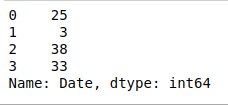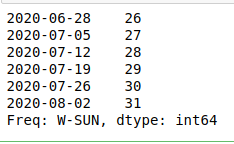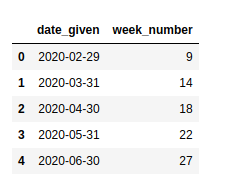Related Articles

# Extract week number from date in Pandas-Python

• Last Updated : 10 Jul, 2020

Many times, when working with some data containing dates we may need to extract the week number from a particular date. In Python, it can be easily done with the help of pandas.

Example 1:

 `# importing pandas as pd``import` `pandas as pd `` ` `# creating a dictionary containing a date``dict` `=` `{``'Date'``:[``"2015-06-17"``]}`` ` `# converting the dictionary to a dataframe``df ``=` `pd.DataFrame.from_dict(``dict``)`` ` `# converting the date to the required format``df[``'Date'``] ``=` `pd.to_datetime(df[``'Date'``], errors ``=``'coerce'``)``df.astype(``'int64'``).dtypes`` ` `# extracting the week from the date``weekNumber ``=` `df[``'Date'``].dt.week`` ` `print``(weekNumber)`

Output:

```0    25
Name: Date, dtype: int64```

Example 2: We can also do the same for multiple dates by adding more dates in the ‘Date’ object.

 `# importing pandas as pd``import` `pandas as pd `` ` `# creating a dictionary containing a date``dict` `=` `{``'Date'``:[``"2020-06-17"``, ``"2020-01-14"``, ``                ``"2020-09-20"``, ``"2020-08-15"``]}`` ` `# converting the dictionary to a ``# dataframe``df ``=` `pd.DataFrame.from_dict(``dict``)`` ` `# converting the date to the required ``# format``df[``'Date'``] ``=` `pd.to_datetime(df[``'Date'``],``                            ``errors ``=``'coerce'``)``df.astype(``'int64'``).dtypes`` ` `# extracting the week from the date``weekNumber ``=` `df[``'Date'``].dt.week`` ` `print``(weekNumber)`

Output:Example 3: Extracting week number from dates for multiple dates using date_range() and to_series().

• pandas.data_range(): It generates all the dates from the start to end date

Syntax:

pandas.date_range(start, end, periods, freq, tz, normalize, name, closed)

• pandas.to_series(): It creates a Series with both index and values equal to the index keys.

Syntax:

`Index.to_series(self, index, name)`
 `# importing pandas as pd``import` `pandas as pd `` ` `# generating all dates in given range``# with increment by days``allDates ``=` `pd.date_range(``'2020-06-27'``, ``'2020-08-03'``, freq ``=``'W'``)`` ` `# converting dates to series``series ``=` `allDates.to_series()`` ` `series.dt.week`

Output:Example 4: In this example, we’ll be using pandas.Series() to generate dates and use a different way to convert the series to the dataframe.

pandas.Series(): Used to create a one-dimensional ndarray with axis labels.
Syntax:

`pandas.Series(data, index, dtype, name, copy, fastpath)`
 `# importing pandas as pd``import` `pandas as pd `` ` `# generating the series``dates ``=` `pd.Series(pd.date_range(``'2020-2-10'``,``                                ``periods ``=` `5``,``                                ``freq ``=``'M'``))`` ` `# converting to dataframe``df ``=` `pd.DataFrame({``'date_given'``: dates})`` ` `# extracting the week number``df[``'week_number'``] ``=` `df[``'date_given'``].dt.week`` ` `df`

Output:Attention geek! Strengthen your foundations with the Python Programming Foundation Course and learn the basics.

To begin with, your interview preparations Enhance your Data Structures concepts with the Python DS Course. And to begin with your Machine Learning Journey, join the Machine Learning – Basic Level Course

My Personal Notes arrow_drop_up